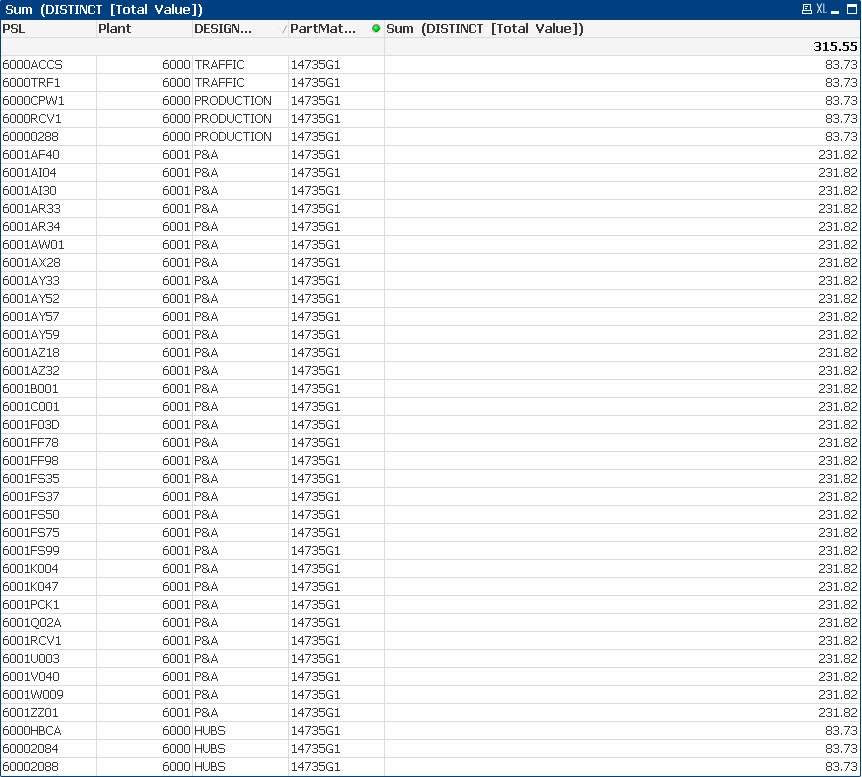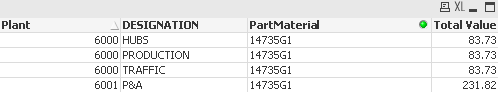New to QlikView

Discussion board where members can get started with QlikView.

AnnouncementsNot applicable

Getting a Total based on Multiple Fields

My current data set is this table here:I want to pretty much condense the data down to something similar to this:I want to sum the distinct [Total Value], based on the Plant and DESIGNATION.

For example:

Plant 6000, DESIGNATION TRAFFIC has two [Total Value]'s of 83.73. Which would equal a sum of 83.73.

Plant 6000, DESIGNATION PRODUCTION has three [Total Value]'s of 83.73. Which would equal a sum of 83.73.

Plant 6000, DESIGNATION HUBS has three [Total Value]'s of 83.73. Which would equal a sum of 83.73.

Now if Plant 6000, DESIGNATION TRAFFIC were to have multiple [Total Value]'s of let's say for example 83.73 and 116.27; I would want the sum to be 200. This is because the two distinct values of 83.73 and 116.27 from Plant 6000, DESIGNATION TRAFFIC, sum up to be 200.

Next, I want the sum of those three summations, so I could conclude that Plant 6000 has a conclusive total value of 251.19.

What expression would I have to use to accomplish this? I have been trying out different set analysis statements to accomplish this, but to no avail.

Thank you!

Tags (2)
1 Solution

Accepted SolutionsMVP

Re: Getting a Total based on Multiple Fields

May be like this

Sum(Aggr(Sum(DISTINCT [Total Value]), Plant, DESIGNATION))

3 Replies
Valued Contributor

Re: Getting a Total based on Multiple Fields

in the front end

straight  or pivot table

Dimension

PLANT

DESIGNATION

expression

Max(Value)MVP

Re: Getting a Total based on Multiple Fields

May be like this

Sum(Aggr(Sum(DISTINCT [Total Value]), Plant, DESIGNATION))Not applicable

Re: Getting a Total based on Multiple Fields

Hot diggity dang. It worked like a charm. Thank you!!